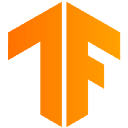# RLlib: Scalable Reinforcement Learning¶

RLlib is an open-source library for reinforcement learning that offers both high scalability and a unified API for a variety of applications. RLlib natively supports TensorFlow, TensorFlow Eager, and PyTorch, but most of its internals are framework agnostic.To get started, take a look over the custom env example and the API documentation. If you’re looking to develop custom algorithms with RLlib, also check out concepts and custom algorithms.

Tip

## RLlib in 60 seconds¶

The following is a whirlwind overview of RLlib. For a more in-depth guide, see also the full table of contents and RLlib blog posts. You may also want to skim the list of built-in algorithms. Look out for theandicons to see which algorithms are available for each framework.

### Running RLlib¶

RLlib has extra dependencies on top of ray. First, you’ll need to install either PyTorch or TensorFlow. Then, install the RLlib module:

pip install 'ray[rllib]'  # also recommended: ray[debug]


Then, you can try out training in the following equivalent ways:

rllib train --run=PPO --env=CartPole-v0  # -v [-vv] for verbose,
# --eager [--trace] for eager execution,
# --torch to use PyTorch

from ray import tune
from ray.rllib.agents.ppo import PPOTrainer
tune.run(PPOTrainer, config={"env": "CartPole-v0"})  # "log_level": "INFO" for verbose,
# "framework": "tfe"/"tf2" for eager,
# "framework": "torch" for PyTorch


Next, we’ll cover three key concepts in RLlib: Policies, Samples, and Trainers.

### Policies¶

Policies are a core concept in RLlib. In a nutshell, policies are Python classes that define how an agent acts in an environment. Rollout workers query the policy to determine agent actions. In a gym environment, there is a single agent and policy. In vector envs, policy inference is for multiple agents at once, and in multi-agent, there may be multiple policies, each controlling one or more agents:Policies can be implemented using any framework. However, for TensorFlow and PyTorch, RLlib has build_tf_policy and build_torch_policy helper functions that let you define a trainable policy with a functional-style API, for example:

def policy_gradient_loss(policy, model, dist_class, train_batch):
logits, _ = model.from_batch(train_batch)
action_dist = dist_class(logits, model)
return -tf.reduce_mean(
action_dist.logp(train_batch["actions"]) * train_batch["rewards"])

# <class 'ray.rllib.policy.tf_policy_template.MyTFPolicy'>
MyTFPolicy = build_tf_policy(
name="MyTFPolicy",


### Sample Batches¶

Whether running in a single process or large cluster, all data interchange in RLlib is in the form of sample batches. Sample batches encode one or more fragments of a trajectory. Typically, RLlib collects batches of size rollout_fragment_length from rollout workers, and concatenates one or more of these batches into a batch of size train_batch_size that is the input to SGD.

A typical sample batch looks something like the following when summarized. Since all values are kept in arrays, this allows for efficient encoding and transmission across the network:

{ 'action_logp': np.ndarray((200,), dtype=float32, min=-0.701, max=-0.685, mean=-0.694),
'actions': np.ndarray((200,), dtype=int64, min=0.0, max=1.0, mean=0.495),
'dones': np.ndarray((200,), dtype=bool, min=0.0, max=1.0, mean=0.055),
'new_obs': np.ndarray((200, 4), dtype=float32, min=-2.46, max=2.259, mean=0.018),
'obs': np.ndarray((200, 4), dtype=float32, min=-2.46, max=2.259, mean=0.016),
'rewards': np.ndarray((200,), dtype=float32, min=1.0, max=1.0, mean=1.0),
't': np.ndarray((200,), dtype=int64, min=0.0, max=34.0, mean=9.14)}


In multi-agent mode, sample batches are collected separately for each individual policy.

### Training¶

Policies each define a learn_on_batch() method that improves the policy given a sample batch of input. For TF and Torch policies, this is implemented using a loss function that takes as input sample batch tensors and outputs a scalar loss. Here are a few example loss functions:

RLlib Trainer classes coordinate the distributed workflow of running rollouts and optimizing policies. They do this by leveraging Ray parallel iterators to implement the desired computation pattern. The following figure shows synchronous sampling, the simplest of these patterns:Synchronous Sampling (e.g., A2C, PG, PPO)

RLlib uses Ray actors to scale training from a single core to many thousands of cores in a cluster. You can configure the parallelism used for training by changing the num_workers parameter. Check out our scaling guide for more details here.

### Application Support¶

Beyond environments defined in Python, RLlib supports batch training on offline datasets, and also provides a variety of integration strategies for external applications.

### Customization¶

RLlib provides ways to customize almost all aspects of training, including the environment, neural network model, action distribution, and policy definitions: